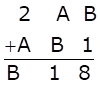Solve Cryptarithms:Asked by Sakshi | 1 year ago |  82

##### Solution :-

Firstly let us solve for unit’s place,

B + 1 = 8

B = 7

Now let us solve for ten’s place,

A + B = 1

A + 7 = 1

A = -6 which is not possible.

Hence, A + B > 9

We know that now there should be one carry in hundred’s place and so we need to subtract 10 from ten’s place,

i.e., A + B – 10 = 1

A + 7 = 11

A = 11-7 = 4

Now to check whether our values of A and B are correct, we should solve for hundred’s place.

2 + A + 1 = B

2 + 4 + 1 = 7

7 = 7

i.e., RHS = LHS

A = 4 and B = 7

Answered by Aaryan | 1 year ago

### Related Questions

#### Which of the following numbers are divisible by 11

Which of the following numbers are divisible by 11:

(i) 10835

(ii) 380237

(iii) 504670

(iv) 28248

#### In each of the following replace * by a digit so that the number formed is divisible by 11:

In each of the following replace * by a digit so that the number formed is divisible by 11:

(i) 64 × 2456

(ii) 86 × 6194

#### In each of the following replace * by a digit so that the number formed is divisible by 6:

In each of the following replace * by a digit so that the number formed is divisible by 6:

(i) 97 × 542

(ii) 709 × 94

#### In each of the following replace × by a digit so that the number formed is divisible by 9:

In each of the following replace × by a digit so that the number formed is divisible by 9

(i) 49 × 2207

(ii) 5938 × 623